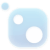# Top 20 NuGet descent Packages

SimpleML provides implementations of basic machine learning algorithms.
Collaborative Filtering Recommender
This library aims to implement different mathematical optimization algorithms, such as regular and conjugate gradient descent.## The C++ Mathematical Expression Toolkit Library (ExprTk)

The C++ Mathematical Expression Toolkit Library (ExprTk) is a simple to use, easy to integrate and extremely efficient run-time mathematical expression parsing and evaluation engine. The parsing engine supports numerous forms of functional and logic processing semantics and is easily extendible.
The C++ Mathematical Expression Toolkit Library (ExprTk) Extra Examples
Machine Learning library for .NET Core
The Spart library is an object oriented recursive descent parser generator framework implemented in C#.